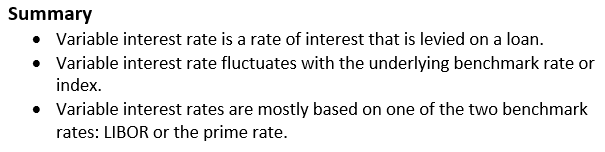# Variable Interest Rate

• Updated on

What is Variable Interest Rate?

Variable interest rate refers to the rate of interest that varies with the change of index and benchmark interest rate over a period. Variable interest rate is also known as floating or adjustable interest rate as it fluctuates with the variation in index or rate of underlying benchmark such as prime rate.Understanding Variable Interest Rate

A variable interest rate is a rate of interest that charged to borrower on his/her loan with the fluctuating interest rate.  Loans have different terms and conditions; some include fixed rate of interest that means borrower has to pay same rate throughout the life of loan and some includes variable rate of interest that means interest may fluctuate throughout loan’s life.

A variable interest rate fluctuates with benchmark rate or index. The index or underlying benchmark rate mainly based on LIBOR (London Interbank Offered Rate) and federal funds rate and depends on the type of the loan or security of variable interest rate. If the index or benchmark rate falls or increases, interest rate of a borrower will change too. With the fall in rate of underlying benchmark or index, borrower’s interest payment decreased. And if the rate of underlying benchmark or index rises, borrower’s interest payment rises too.

How variable interest rate works?

Variable interest rate is usually determined at the time of approval of loan, although interest rate will changes over time. The variable rate depends on two components: a credit-based margin and an index. A credit-based margin is determined by lender and an index is available publicly. Variable interest rate is lower in starting than the fixed rate interest rate that makes a loan more affordable.

Variable interest rates mostly based on one of two benchmark rates: LIBOR and the prime rate. These two benchmarks help financial institutes to decide the price of money. London Interbank Offered Rate, or LIBOR, and the prime rate used by the lenders as the baselines for variable rate loans. Lenders add margin on the top of the benchmark rate in order to get the rate received by borrower. The margin and interest rate received by the borrower on a variable rate loan is depends on loan product and the credit score of a borrower. For instance, credit card companies determine the interest rates for next month by using the prime rate that is recorded in the Wall Street Journal at the end of a month.

What is the difference between variable and fixed interest rate?

Fixed interest rate refers to the interest rate that remains fixed throughout the life of the loan. The interest rate will not change due to market changes; it won’t change till the ending of the loan terms. Unlike fixed interest rate, variable interest rate fluctuates with the changes in market including index and benchmark interest rate.

Fixed interest rates are usually higher in the beginning of the loan in the comparison of variable interest rates; variable interest rate is lower in starting that makes a loan more affordable. In variable interest rates, monthly payments are not fixed it may change with the fluctuation in the market. Wherein fixed interest rates, monthly payments are fixed and remain the same till the life of the loan.

Fixed interest rate is not dependent on any financial index and it is the rate provided by the lender at up front based on the credit score of a borrower, current economic conditions, and other related factors. In fixed interest rate, borrower knows the total loan payment costs. On the contrary, total loan payment costs are uncertain.

What are the merits of Variable Interest Rate?

One of the biggest advantages of variable interest rate for the borrower is that the rate fluctuates throughout the duration of loan, which means if the interest rate falls with the fall in index or underlying benchmark rate, borrower’s interest payment will fall to. Borrower has to pay less interest payment on the loan.

Talking about lender’s perspective, lender may adjust the interest rate upward with the changes of market, while fixed interest rate will remain same and does not change with the market change. The benefits enjoyed by both the borrower and lender are:

• Variable interest rates are generally lower than fixed interest rates.
• Borrower gets benefit if interest rate falls.
• Lender gets benefit if interest rate rises.
• Variable interest rate is lower in starting that makes a loan more affordable.

What are the demerits of Variable Interest Rate?

Variable interest rate may affect the borrower’s ability of repaying a loan. If the interest rate will rise due to market changes and with the rise in the underlying benchmark rate or index, Borrower has to pay more interest on loan. If a borrower planning to take loan with variable rate of interest, he/she should go through with all the details, terms and conditions in order to know how often rates can fluctuate and what can be the highest possible payment. These are the following point which may considered as demerits of variable interest rate.

• Sometimes variable interest rates rise up to the point where the loan is unaffordable for a borrower.
• The uncertainty of variable interest rates makes the repayment cost out of a borrower’s budget.
• Future cash flows are unpredictable due to fluctuations in the interest rate.

##### Top ASX Listed Companies

Definition:
We use cookies to ensure that we give you the best experience on our website. If you continue to use this site we will assume that you are happy with it. OK